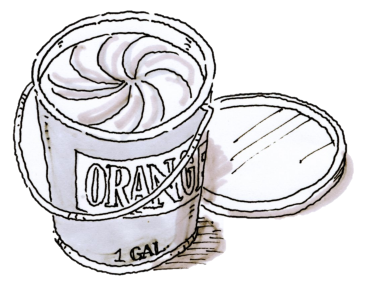### Home > CCAA > Chapter 5 Unit 3 > Lesson CC2: 5.1.2 > Problem5-18

5-18.

A shade of orange paint is made with $5$ parts red paint and $15$ parts yellow paint.

1. What percent of the paint is red?

Convert the information into a fraction. Remember that the "whole" in this case is the total parts of both red and yellow paint.

Use a Giant One to change the fraction to an equivalent fraction with a denominator of $100$.

The numerator is the percentage of red paint.

$25\%$

2. What is the simplified ratio of yellow to red paint?

Convert the information into a fraction. But this time the numerator is the parts of yellow paint and the denominator is the parts of red paint.

Reduce the fraction as much as possible.